JEE  >  Test: Displacement & Velocity - 2

# Test: Displacement & Velocity - 2

Test Description

## 10 Questions MCQ Test JEE Foundation | Test: Displacement & Velocity - 2

Test: Displacement & Velocity - 2 for JEE 2023 is part of JEE Foundation preparation. The Test: Displacement & Velocity - 2 questions and answers have been prepared according to the JEE exam syllabus.The Test: Displacement & Velocity - 2 MCQs are made for JEE 2023 Exam. Find important definitions, questions, notes, meanings, examples, exercises, MCQs and online tests for Test: Displacement & Velocity - 2 below.
Solutions of Test: Displacement & Velocity - 2 questions in English are available as part of our JEE Foundation for JEE & Test: Displacement & Velocity - 2 solutions in Hindi for JEE Foundation course. Download more important topics, notes, lectures and mock test series for JEE Exam by signing up for free. Attempt Test: Displacement & Velocity - 2 | 10 questions in 30 minutes | Mock test for JEE preparation | Free important questions MCQ to study JEE Foundation for JEE Exam | Download free PDF with solutions
 1 Crore+ students have signed up on EduRev. Have you?
Test: Displacement & Velocity - 2 - Question 1

### The displacement-time graph for two particles A and B are straight lines inclined at angles of 300 and 600 with the time axis. The ratio of velocities of VA: VB is:

Detailed Solution for Test: Displacement & Velocity - 2 - Question 1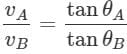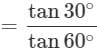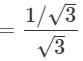Test: Displacement & Velocity - 2 - Question 2

### A 150 m long train is moving with a uniform velocity of 45 km/h. The time taken by the train to cross a bridge of length 850 meters is:

Detailed Solution for Test: Displacement & Velocity - 2 - Question 2

Total distance to be covered for crossing the bridge = length of train + length of bridge
= 150 m + 850 m = 1000 m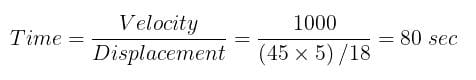Test: Displacement & Velocity - 2 - Question 3

### A particle is constrained to move on a straight-line path. It returns to the starting point after 10 sec. The total distance covered by the particle during this time is 30 m. Which of the following statements about the motion of the particle is true?

Detailed Solution for Test: Displacement & Velocity - 2 - Question 3
• Displacement of the particle will be zero because it comes back to its starting point.
•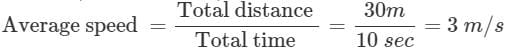Test: Displacement & Velocity - 2 - Question 4

A particle moves along a semicircle of radius 10m in 5 seconds. The average velocity of the particle is :

Detailed Solution for Test: Displacement & Velocity - 2 - Question 4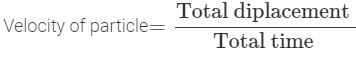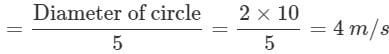Test: Displacement & Velocity - 2 - Question 5

The ratio of the numerical values of the average velocity and average speed of a body is always:

Detailed Solution for Test: Displacement & Velocity - 2 - Question 5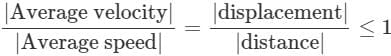Because displacement will either be equal or less than distance. It can never be greater than distance.

Test: Displacement & Velocity - 2 - Question 6

Two boys are standing at the ends A and B of ground where AB = a. The boy at B starts running in a direction perpendicular to AB with velocity v1. The boy at A starts running simultaneously with velocity v and catches the other boy in a time t, where t is:

Detailed Solution for Test: Displacement & Velocity - 2 - Question 6

Let two boys meet at point C after time 't' from the starting.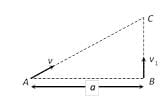Then
AC = vt  and BC = v1t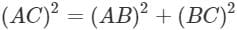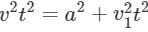By solving we get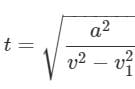Test: Displacement & Velocity - 2 - Question 7

If a car covers 2/5th of the total distance with v1 speed and 3/5th distance with v2 then the average speed is:

Detailed Solution for Test: Displacement & Velocity - 2 - Question 7

Average Speed =
Total Distance Travelled / Total Time Taken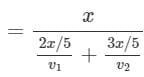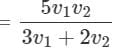Test: Displacement & Velocity - 2 - Question 8

A man walks on a straight road from his home to a market 2.5 km away with a speed of 5 km/h. Finding the market closed, he instantly turns and walks back home with a speed of 7.5 km/h. The average speed of the man over the interval of time 0 to 40 min. is equal to :

Detailed Solution for Test: Displacement & Velocity - 2 - Question 8

A man walks from his home to market with a speed of 5 km/h.
Distance = 2.5 km
Time = Distance / Time
= 2.5 / 5 = 1/2 hr
He returns back with speed of 7.5 km/h in rest of time of 10 minutes.
Distance =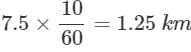So, Average Speed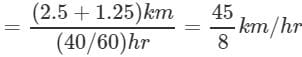Test: Displacement & Velocity - 2 - Question 9

A person travels along a straight road for half the distance with velocity v1 and the remaining half distance with velocity v2.The average velocity is given by:

Detailed Solution for Test: Displacement & Velocity - 2 - Question 9

As the total distance is divided into two equal parts therefore distance averaged speed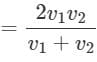Test: Displacement & Velocity - 2 - Question 10

Distance and displacement of a moving object have same magnitude when ______.

Detailed Solution for Test: Displacement & Velocity - 2 - Question 10

The distance and displacement of moving object will have the same magnitude when the object moves along a straight line in the same fixed direction.

## JEE Foundation

99 videos|291 docs|212 tests
 Use Code STAYHOME200 and get INR 200 additional OFF Use Coupon Code
Information about Test: Displacement & Velocity - 2 Page
In this test you can find the Exam questions for Test: Displacement & Velocity - 2 solved & explained in the simplest way possible. Besides giving Questions and answers for Test: Displacement & Velocity - 2, EduRev gives you an ample number of Online tests for practice

## JEE Foundation

99 videos|291 docs|212 tests